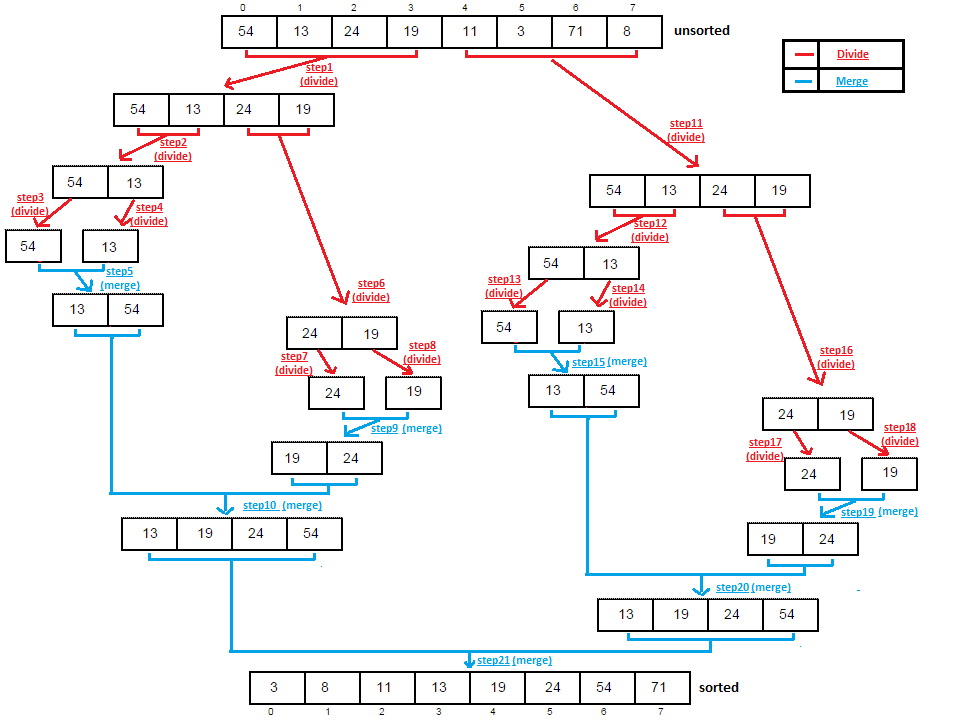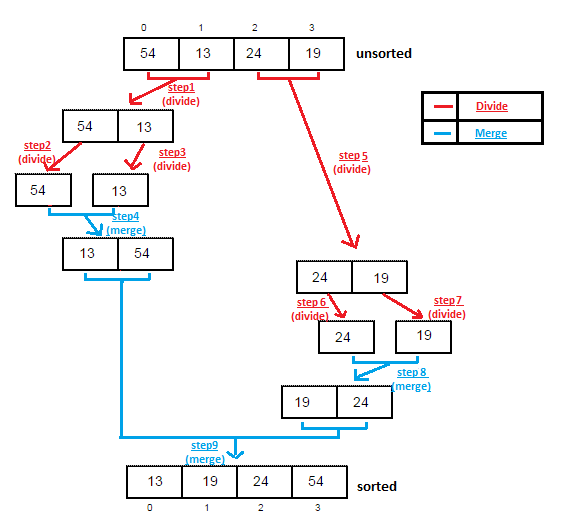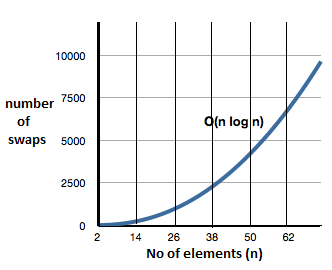### Merge Sort with complexity explanation and diagram in java

Contents of page >
• How Merge sort works?
• Diagram of merge sort in java >
• But how array is divided into halves ?
• Let’s merge sort array of 8 elements using diagram.
• Full Program/SourceCode/Example of Merge Sort in java>
• Complexity of Merge Sort in java >
• Comparisons required in Merge Sort in java >
• Complexity of Merge Sort in graph >How Merge sort works?
Merge sort divides array into half, sort each half, and merge each half to form sorted array.

First, it’s important to understand, how we Merge two sorted arrays.

Diagram of merge sort in java >
Let’s merge sort array of 4 elements in java.Array is divided into two halves with range (0-1) & (2-3)  [in step1 and step2]
we have merged two halves (in step4 & step8) &
finally merged both halves to form sorted array(in step9).

Must read: Bubble Sort, Insertion Sort, QUICKSORT in java.

But how array is divided into halves ?
Array is divided into halves using recursion,

Recursively mergeSort method is called, method calls itself again and again to form smaller ranges until the array is divided into smallest range, than each time it returns larger merged array formed from smaller ranges.

Above array is divided into bottom half (0-1)  [in step1]

range(0-1) is again divided into bottom half(0-0) [in step2]

 But, range(0-0) is a single element only. So, no merging is required.

& upper half(1-1). [in step3]

 Also, range (1-1) is again a single element. So, no merging is required.

Than, merge range(0-1) [in step4], now range (0-1) is sorted in itself.

& upper half(2-3).  [in step5]

range (2-3) is again divided into bottom half (2-2) [in step6]

 But, range(2-2) is a single element only. So, no merging is required.

& upper half(3-3). [in step7]

 Also, range (3-3) is again a single element. So, no merging is required.

Than merge range(2-3) [in step8], now range (2-3) is sorted in itself.

Finally, we merges ranges (0-1) & (2-3) to form larger sorted array [in step9], now we have got sorted array.

We can use Bubble Sort, Selection Sort, Insertion Sort,  quick Sort which may offer you different time complexity.

Let’s merge sort array of 8 elements using diagram.Full Program/SourceCode/Example of Merge Sort in java>
 /** *  write a program to do Merge Sort of array in java. */ public class MergeSortAlgorithmExample {    static int[] ar = { 54, 13, 24, 19, 11, 3, 71, 8 };       /**    * Main method which calls merge sort in java    * @param args    */    public static void main(String[] args) {                     System.out.print("Display array before Merge sorting: "  );           for (int i = 0; i < ar.length; i++)                  System.out.print(ar[i] +" ");                     int[] ar2 = new int[ar.length];           mergeSort(ar2, 0, ar.length - 1);                     System.out.print("\nDisplay array after Merge sort: "  );           for (int i = 0; i < ar.length; i++)                  System.out.print(ar[i] +" ");              }    /*    * method performs merge sort in java.    */    static void mergeSort(int[] workSpace, int lowerBound, int upperBound) {           if (lowerBound == upperBound){                  return;    //no need to sort further.           }else {    // finding mid point                  int midPoint = (lowerBound + upperBound) / 2;                  mergeSort(workSpace, lowerBound, midPoint); // sorting low half                  mergeSort(workSpace, midPoint + 1, upperBound); // sort upper half                  merging(workSpace, lowerBound, midPoint + 1, upperBound);//merging them           }    }          static void merging(int[] ar2, int low, int high, int upperBound) {                     int midPoint = high - 1;           int lowerBound = low;           int n = upperBound - lowerBound + 1;           int i = 0;                     while (low <= midPoint && high <= upperBound)                  if (ar[low] < ar[high])                        ar2[i++] = ar[low++];                  else                        ar2[i++] = ar[high++];                     while (low <= midPoint)                  ar2[i++] = ar[low++];                     while (high <= upperBound)                  ar2[i++] = ar[high++];                     for (i = 0; i < n; i++)                  ar[lowerBound + i] = ar2[i];              } } /*OUTPUT Display array before Merge sorting: 54 13 24 19 11 3 71 8 Display array after Merge sort: 3 8 11 13 19 24 54 71 */

So, we wrote program to do Merge Sort of array in java.

Complexity of Merge Sort in java >
Best,average and worst case >

Best Case :         O(N*logN) , though no of swaps may be 0 (if array is already sorted)
Average Case :  O(N*logN).
Worst Case :      O(N*logN), no of swaps may be very high (if array is reversely sorted)
also no of comparisons may be very high if we are merging two arrays of size i & j. (where i<j) Ex. i may be 2 and j may be 100(so many extra comparisons will be made in that case.).

Comparisons required in Merge Sort in java >

 N (number of elements) O(logN) O(N*logN) 8 3 24 16 4 64 32 5 160 64 6 384 128 7 896 256 8 2048

Complexity of Merge Sort in graph >Summary >
So in this data structure tutorial we learned how array is divided into halves. Merge sort array of 8 elements using diagram. Program/Example of Merge Sort in java. Complexity of Merge Sort in java. Comparisons required in Merge Sort in java. Complexity of Merge Sort in graph.

Having any doubt? or you you liked the tutorial! Please comment in below section.